Disclaimer: This is an example of a student written essay.

Any scientific information contained within this essay should not be treated as fact, this content is to be used for educational purposes only and may contain factual inaccuracies or be out of date.

# Low Altitude Measurement Accuracy Improvement of the Airborne FMCW Radio Altimeters

 ✅ Paper Type: Free Essay ✅ Subject: Engineering ✅ Wordcount: 5729 words ✅ Published: 18th May 2020

Abstract: The manuscript focuses on the analysis of a critical height of radio altimeters that can help at the development of new types of aeronautical radio altimeters with increased accuracy in measuring the low altitudes. Altitude measurement accuracy is connected with a form of processing the difference signal of a radio altimeter which carries information on the measured altitude. The definition of the altitude measurement accuracy is closely linked to the value of a critical height. Modern radio altimeters with digital processing of a difference signal could shift the limit of accuracy towards better values when the basics of the determination of critical height are thoroughly known. The theory, results of the analysis and simulation of dynamic formation and dissolution of so-called stable and unstable height pulses which define the range of critical height are presented in the paper. The theory is supported by a new method of derivation of the basic equation of a radio altimeter based on a critical height. The article supports the new theory of radio altimeters with the ultra-wide bandwidth leading to the increase the accuracy of a low altitude measurement. Complex mathematical analysis of the dynamic formation of critical height, computer simulation of its course supported by the new form of the derivation of the basic equation of radio altimeter guarantee the correctness of the new findings. Application of the presented findings to the aviation practice will contribute to increasing the accuracy of the low altitude measurement from aircraft during its landing and increasing the air traffic safety.

Keywords: FMCW radio altimeter; methodological error; critical height; altitude measurement accuracy; height pulses; ultra-wide bandwidth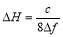, (1)

where c is the speed of light, Δf is the frequency deviation.

The range of critical height for recent radio altimeters (corresponding with measurement accuracy) has settled on the value of 0.75 m. The manuscript deals with the principle of the formation of critical height and suggestions on how to decrease its value to increase the altitude measurement accuracy [5,6].

2. Difference signal of FMCW radio altimeter

From a mathematical analysis of the correlation amplitude ratios of the frequency-modulated transmitting signal UT and time delay of the received signal UR, it is possible to derive the time course of the amplitude of the difference signal Ud[5,7,8].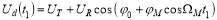, (2)

where φ0 and φM are the initial and variable phase of the difference signal, ΩM is the angular frequency modulation of the signal, t1 is the time course of the difference signal, t1 = (tτ/2).

This signal allows the formation of so-called unstable height pulses Nu. They alternately arise and disappear when the amplitude of the difference signal passes through zero [3,9,10]. Defining the condition of the formation and dissolution of the unstable height pulses allows determining the rise HF and extinction HE heights of the individual pulses Nu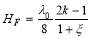,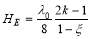, (3)

where λ0 is the carrier wavelength, k is the sequence number of the pulse height, ξ is the relative value of the frequency deviation, ξ = Δf/f0.

Then two heights behind forming pulses make it possible to define the height range of the formation of individual pulses F, and from two heights of the following dissolution pulses to define the height range of dissolving pulses E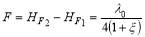,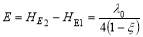. (4)

A height range of the duration of different unstable height pulse S is determined as the difference of height between the dissolution and formation of any height pulse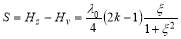. (5)

Equation (4) shows us that the height range of gradual formation of different unstable height pulses is constant and smaller than the value of λ0/4 at any measured height. Thus, it is possible to state that F1 = F2 = F3 = …. At the same time, it can be seen that the height range of dissolution of unstable pulses E is also constant but bigger than the value of λ0/4 at any measured height. So it is also possible to state that E1 = E2 = E3 = …. Equation (5) also indicates that the height range of duration of different unstable height pulses constantly grows with the increase of consequence number of pulse k as a result of the increase of the value of measured altitude. And so, it is possible to state that S1 < S2 < S3 < … [3,11].

Gradual increase of the height range of the duration of different unstable pulses together with growing measured altitude cause that more and more of those pulses at the same height overlap mutually (Figure 1 and 2) .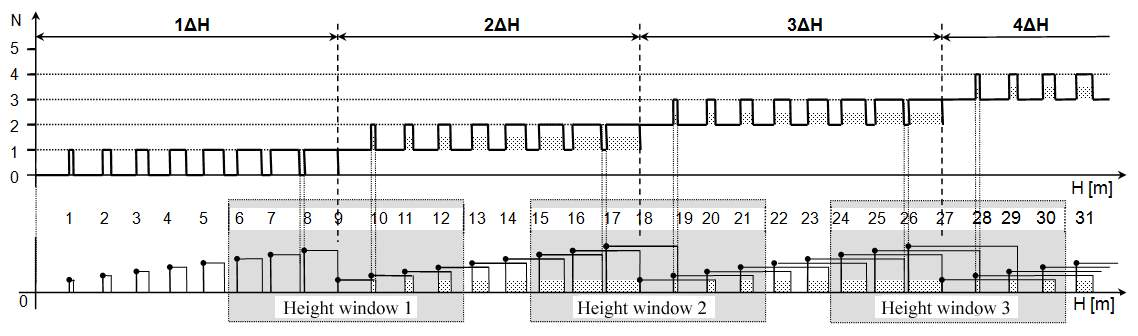Figure 1. Height dependence of the formation and dissolution of unstable height pulses.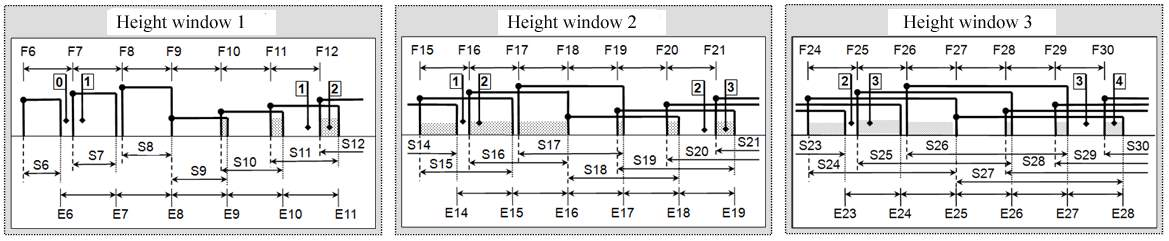Figure 2. Height ranges and windows in the area of mutual overlapping of unstable height pulses.

3. Simulation of Height Pulses Creation

Based on the above-defined theory the simulation program for making the stable Nc and unstable Nu height pulses of the radio altimeter have been created. The simulation results at the input parameters equal to the value of the current radio altimeters are shown in Figure 3. The simulation is realized in a height range from 0 m to 2 m with a mean value of the height of 1 m. In Figure 3, we can see that the first critical height value ends at 0.75 m height level and the second one ends at 1.5 m. Obviously, the methodic error of the radio altimeter (0.75 m) is the same as given by the radio altimeter manufacturers.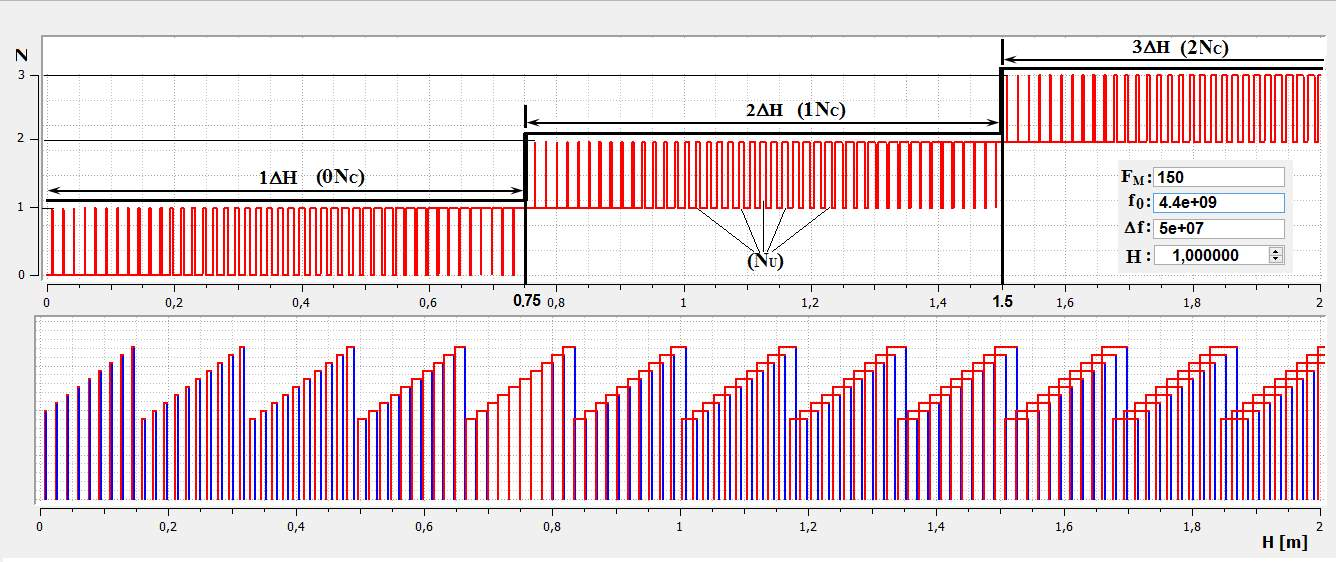Figure 3. Simulation of the formation of stable and unstable height pulses.

The upper part of Figure 3 presents the height dependence of the occurrence of stable pulses and also the height dependence of the formation and dissolution of the unstable pulses with increasing height. In the 2-m height range, three critical heights are simulated, that corresponds to the two ranges of the existence of the stable pulses 2Nc. There is no stable pulse in the first range (0Nc in1ΔH in Figure 3). A larger number of unstable pulses is simulated in the same 2-m height range.

If you need assistance with writing your essay, our professional essay writing service is here to help!

The bottom part of Figure 3 presents height ranges of the duration of different pulses S. At the critical height of 1ΔH, the height ranges of the duration of unstable height pulses are so small that they do not overlap and an alternation of zero and first unstable pulses (0 – 1) occurs at the given critical height. At all the following critical heights, the height ranges of the duration of unstable height pulses are so big that they mutually overlap. The number of permanently existing unstable pulses is determined by the consequence number of a stable height pulse. At least one unstable pulse exists at each section of the height within the range of 2ΔH. It means that one stable pulse exists at the height of alternation of the first and second unstable pulses (1 – 2) within the given range. Similarly, two unstable pulses exist permanently within the range of 3ΔH in each section of the height. It means that there are two stable pulses.

Closer analysis of this phenomenon can be observed that the process of formation and dissolution of the unstable height of pulses is not chaotic but has regularity. The regularity of formation and dissolution of height pulses is related to the change in the measured height but their number in the range of critical height corresponds to the technical parameters of the radio altimeter [9,10].

4. Number of Unstable Pulses in Range of Critical Height

It is obvious from Figures 1 and 2 presenting the height dependence of the formation of height pulses, as well as from Figure 3 showing the results of the simulation of stable and unstable height pulses that the higher the number of unstable pulses is in the range of critical height the smaller is the precision of height measurement.

For the logical explanation of reasoning and determination of the number of unstable pulses in the range of critical height, it is necessary to move from the frequency area into the area of wavelengths. This thought is based on the generally known facts about the principle of the operation of FMCW radio altimeters presented in Figure 4.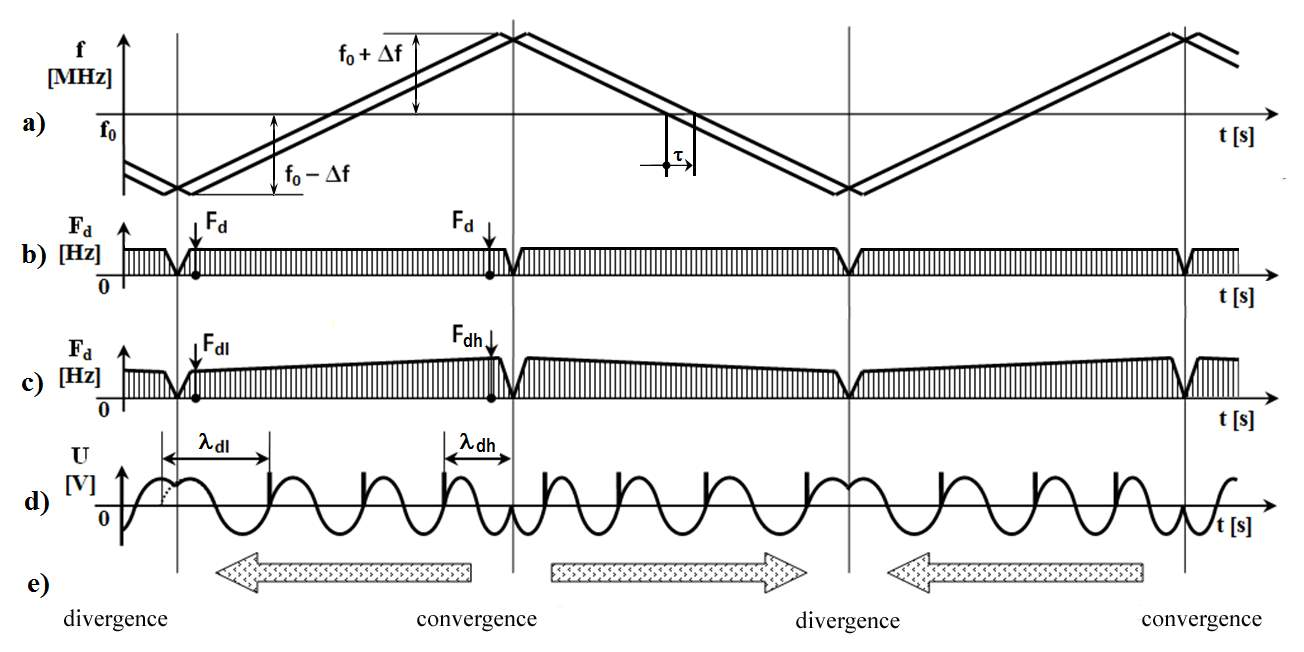Figure 4. Formation of differential signal for determination of the number of unstable height pulses.

Based on principles that if the frequency modulation of the transmitted signal within whole range of ±Δf is linear (Figure 4a) and the time delay of received signal during whole modulation period by value τ is also linear, the immediate value of differential frequency is constant during whole time of the modulation period (Figure 4b). The areas of changing of the direction of frequency deviation in the areas of the maximum (f0 + Δf)max and minimum (f0 – Δf)min transmitted frequency are not considered.

However, the actual situation with the formation of the differential signal is different. The difference in frequency is not formed as a simple mathematical difference but as interference of transmitted λT and received λR wavelengths. Then, the wavelength of carrying signal is minimal λ0min in the case of maximum transmitted frequency (f0 + Δf)max, and vice versa, the wavelength of carrying signal is maximal λ0max at minimum transmitted frequency (f0 – Δf)min. With the equivalent time delay τ of the received signal within the whole modulation period but at the different wavelengths λ0min and λ0max, the value of difference frequency (interference) is not formed by the same way. At the wavelength of λ0min, the interference is formed more often and the value of the higher difference frequency Fdh is higher and vice versa at the wavelength λ0max the interference is formed less frequently and the value of the lower difference frequency Fdl is smaller. Considering the presented phenomenon, the differential frequency is the same within the whole range of the modulation period (Figure 4c).

The above-presented theory is confirmed by the observation of realistic display of the difference signal of a radio altimeter on an oscilloscope. In the higher frequency area f0max and lower wavelength λ0min, the higher differential frequency with the lower wavelength λdh will be formed with a higher number of the height pulses N. In the lower frequency area f0min and the higher wavelength λ0max, the lower difference frequency Fdl with the higher wavelength λdl will be formed with a lower number of the height pulses N (Figure 4d).

As a result of such unbalanced formation of differential frequency, and fluent increase of measured height, height pulses on the display of the oscilloscope will shift from the areas with the higher number of pulses into the area with the lower number of pulses. The presented shift of the periods of difference frequency and relevant height pulses is shown in Figure 4e.

Determination of the number of unstable pulses within the range of critical height ΔH is considered for a particular case of a radio altimeter with the carrier frequency of f0 = 4 400 MHz, the modulation frequency of FM = 150 Hz, and the frequency deviation of ±Δf = 25 MHz. In this case we have

f0max = 4400 MHz + 25 MHz = 4425 MHz  λ0min = 67.797·10-3 m,

f0 = 4400 MHz  λ0= 68.182·10-3 m,

f0min = 4400 MHz – 25 MHz = 4375 MHz  λ0max = 68.571·10-3 m.

Then, the difference of wavelengths can be defined

λmax – λmin = 68.571 m – 67.797 m = 0.774·10-3m.

The calculated value expresses the height range of the formation of an unstable height pulse. Half value of the number of the unstable height pulses within half a period of λ0/2 of a carrier frequency f0 presents the number of unstable pulses within the critical height ΔH. Thus, in the case of considered ratio altimeter, the number of unstable pulses is 44.

Figure 5 shows the results of the simulation of the formation of the number of unstable height pulses within the critical height ΔH for the particular type of a radio altimeter.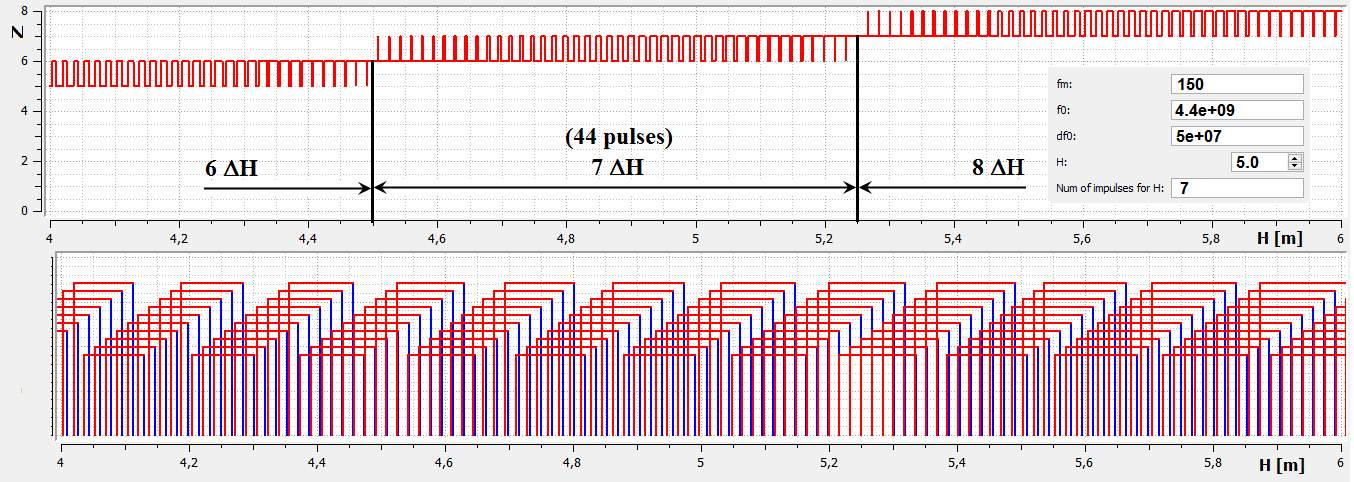Figure 5. Simulation of number of unstable height pulses for the considering radio altimeter.

5. Number of Stable Pulses in Range of Critical Height

A recent method of increasing the accuracy of altitude measurement by the FMCW radio altimeters is based on the increase of frequency deviation with simultaneous increase of the carrier signal frequency. However, the recent radio altimeters use the carrier frequency of 4.4 GHz with the frequency deviation of ±25 MHz (overall bandwidth of 50 MHz) and they can provide the measurement accuracy of ±0.75 m.

Our academic experts are ready and waiting to assist with any writing project you may have. From simple essay plans, through to full dissertations, you can guarantee we have a service perfectly matched to your needs.

It is necessary to increase the carrier frequency with the increase of the frequency deviation due to the requirement for balanced frequency transmission characteristics of the high-frequency circuits as well as active and passive elements of antennas. To increase the accuracy of the altitude measurement in twice (to the value of ±0.375 m) by the same way, the value of the frequency deviation also should be increased in twice (to the value of ±50 MHz that, in fact, corresponds to the overall bandwidth of 100 MHz) with the simultaneous increase of the carrier frequency approximately to 10 GHz.

Based on the above-analyzed theory, it is possible to avoid the problem of the necessity to use a higher carrier frequency of 10 GHz through the use of two parallel high-frequency channels at the original carrier frequency of 4.4 GHz. To create a performance signal, the frequency multipliers are commonly used in radio altimeters nowadays.

In such a way, a basic generator forms the frequency modulated signal with the center frequency of 2200 MHz with the original frequency deviation ±Δf1 = ±25 MHz. Then, the signal is divided by two frequency band-passes into two individual high-frequency channels. Each channel forms its own frequency deviation; the first channel has +Δf1 from 2200 to 2225 MHz, and the second channel has –Δf1  from 2175 to 2200 MHz.

Next, the frequency is independently multiplied two times into the value with the extreme frequency deviation of ±Δf2 = ±50 MHz in each channel; the first channel has +Δf2 from 4400 to 4450 MHz, and the second channel has –Δf2  from 4350 to4400 MHz (Figure 6). Each channel would have only such a bandwidth that is in commonly use, i.e. 50 MHz. At the same time, each channel is tuned in frequency in a different band but the overall bandwidth is 100 MHz. The particular circuit connection of the radio altimeter with extreme bandwidth, which would comply with the above-presented condition, can be achieved by several solutions [13–16].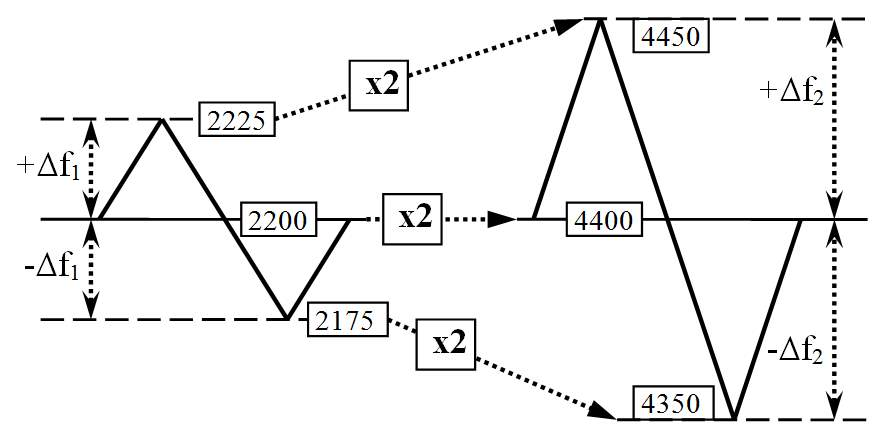Figure 6. Principle of radio altimeter with extreme bandwidth.

Based on the presented background, parameters of the critical height for a radio altimeter with extreme bandwidth have been analyzed with the help of simulation. The parameters of such a radio altimeter are as the following: the carrier frequency is 4400 MHz, the modulation frequency is 150 Hz, and the frequency deviation is 50 MHz, which in sum gives extreme bandwidth of 100 MHz (Figure 7).

From the viewpoint of altitude measurement precision, the results for the radio altimeter with extreme bandwidth are:

a) critical height has the value of 0.375 m;

b) methodological error of the radio altimeter is ±0.375 m;

c) simulated values are at the height of cca 5 m: 4.5 m, 4.875 m, and 5.25 m, respectively, for the bottom, mean, and upper values;

d) number of simulated unstable pulses subtracted within the range of the critical height is 22;

e) number of calculated unstable pulses within range of critical height is also 22.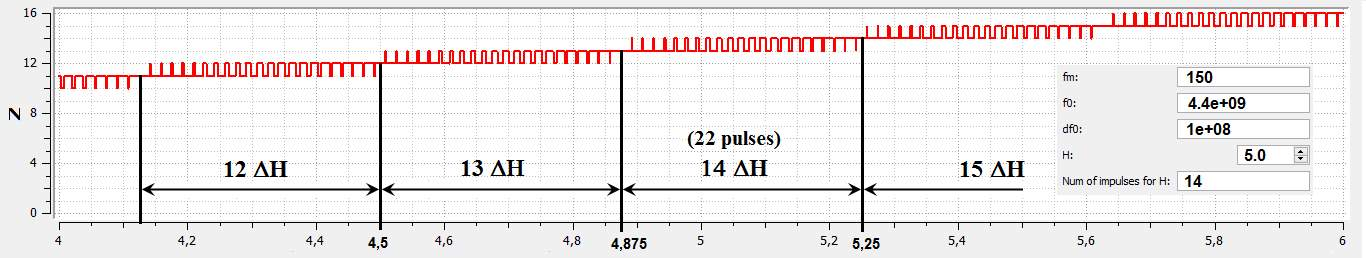Figure 7. Unstable height pulses of radio altimeter with extreme bandwidth.

Based on the theory presented in Section 4, the relation for determination of the number of unstable pulses within the range of critical height has been derived as a part of the research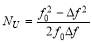. (6)

From the above-presented results, it is possible to conclude that using the double value of the total frequency deviation of 100 MHz at the carrier frequency of 4.4 GHz (a total frequency deviation of 50 MHz is recently used) it is possible to increase the precision of height measurement in twice. In this case, critical height is 0.375 m, and it can be obtained by the formation of 22 unstable height pulses only when one stable pulse is formed. The unstable and stable pulses thought can be supported by the derivation of a basic equation of a radio altimeter from the height pulses formation .

The basic equation of a radio altimeter defines the relation between the value of differential frequency and output information about measured altitude, which is based on the basic parameters of a radio altimeter. Generally, known derivation comes out of the immediate difference of the frequencies of transmitted and received signals, proportionally to the time delay of the received signal, which depends on the measured altitude. A different method for a definition of a basic equation of a radio altimeter, based on critical height has been derived during the research connected with the problem presented in the paper.

As it has been presented in the manuscript, a certain number of stable height pulses, shaped during the time of one modulation period TM corresponds to the measured altitude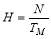. (7)

Formation of only one stable height pulse during the time of one modulation period corresponds to the height change within the range of critical height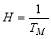. (8)

The following proportion can be obtained from Equations (7) and (8)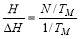. (9)

Replacing the modulation period by the modulation frequency in Equation (9) we have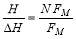. (10)

The product of NFM in Equation (10) presents the difference frequency, which is proportional to the altitude, and so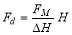. (11)

Substitution of the critical height in Equation (11) from Equation (1) produces the basic equation of a radio altimeter presented in a completely different way from the generally known one, with the use of geometric similarity of triangles [18–20]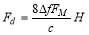. (12)

This fact proves all the above presented theoretical consideration allowing increasing the accuracy of altitude measurement by a radio altimeter.

6. Discussion and Conclusions

We have performed the analysis of the formation of the range of critical height of a radio altimeter methodological error when measuring the altitude. The analysis has been performed from the viewpoint of determination of the number of unstable height pulses within the range of critical height. The manuscript focuses on the formation of unstable pulses within the range of critical height and explains the patterns between formation and dissolution of height pulses. As generally known, unstable pulses do not carry the information about measured altitude; they are not paid enough attention. Some works even consider the chaotic formation of the number of such pulses. Even though the unstable pulses do not determine measured altitude, and they have a critical influence on the precision of the height measurement.

Precise altitude measuring relates to the method of difference signal processing. The presented analysis supported by simulation has confirmed the applicability of the basic idea of processing of the differential signal in the form of creation of stable and unstable pulses.

The process of the determination of the number of unstable pulses within the range of critical height outlines a different view of the basic principle of a radio altimeter (shown in Figure 4c). An original and exact process of the determination of the number of unstable height pulses within the range of critical height for the recent radio altimeters is also presented. This method of calculation has logically leaded out from Equation (6), which has successfully been verified in this manuscript for a different type of a radio altimeter.

The unique solution is based on the decrease of the value of the critical height by the decrease of the number of unstable pulses that results to the double (extreme) increasing the frequency deviation at the original carrier frequency for the recent radio altimeters. In this way, the altitude measurement precision increases from the original value of ±0.75 m to the lower in twice value of ±0.375 m without the need of increasing the carrier frequency (in twice). The correctness of this theory is highlighted by the new way of the derivation of the basic radio altimeter equation with the use of a critical height parameter. The results presented in the paper can be used at the design of new radio altimeters with increased accuracy of the low altitude measurement.

Author Contributions: Conceptualization, J.L.; methodology, J.L.; software, M.Č. and P.K.; validation, M.Č., A.N. and J.G.; formal analysis, J.L., A.N. and J.G.; investigation, J.L., A.N. and J.G.; resources, J.L. and P.K.; data curation, M.Č. and P.K.; writing—original draft preparation, J.L.; writing—review and editing, J.L., A.N. and P.K.; visualization, M.Č. and P.K.; supervision, J.L. and A.N.; project administration, J.G.; funding acquisition, J.G.

Funding: Slovak authors J.L., P.K., M.Č., and J.G. has been supported by the Science Grant Agency of the Ministry of Education Science, Research, and Sport of the Slovak Republic, under contract No. 1/0772/17.

Acknowledgments: A.N. wishes to express his sincere appreciation to the University of Malaga for the provided opportunities during his exchange visit.

Conflicts of Interest: The authors declare no conflict of interest.

References

1. Nebylov, A.V. Aerospace Sensors; Momentum Press: New York, NY, USA 2013; p. 348, doi:10.5643/9781606500613, ISBN 1-60650-059-7.
2. Kelemen, M., Szabo, S., Pedagogical Research of Situational Management in Aviation Education and Forensic Investigation of Air Accidents: Knowledge of Aircraft Operation and Maintenance; Collegium Humanum: Warsaw, Poland, 2019; p. 144, ISBN 978-83-952951-1-9.
3. Labun, J.; Adamčík, F.; Piľa, J.; Madarász, L. Effect of the measured pulses count on the methodical error of the air radio altimeter. Acta Polytech. Hung. 2010, 7, 41–49.
4. Soták, M.; Labun, J. The new approach of evaluating differential signal of airborne FMCW radar-altimeter. Aerosp. Sci. Technol. 2012, 17, 1–6, doi:10.1016/j.ast.2011.02.007.
5. Stove, A.G. Linear FMCW radar techniques. IEE Proc. F (Radar Signal Process.) 1992, 139, 343–350, doi:10.1049/ip-f-2.1992.0048.
6. Skolnik, M.I. Introduction to Radar Systems, 2rd ed.; McGraw Hill Book Company: Singapore, 1981; p. 581, ISBN 0-07-057909-1.
7. Taylor, J. Aircraft Operation of Radio Altimeters. In Proceedings of the 24th Meeting of Working Group F, Aeronautical Communications Panel (ACP), Paris, France, 17–21 March 2011.
8. Alivizatos, E.G.; Petsios, M.N.; Uzunoglu, N.K. Architecture of a multistatic FMCW direction-finding radar. Aerosp. Sci. Technol. 2008, 12, 169–176, doi:10.1016/j.ast.2007.05.001.
9. Baskakov, A.I.; Komarov, A.A.; Mikhailov, M.S.; Ruban, A.V. Modeling of the methodical errors of high-precision aircraft radar altimeter operating above the seasurface at low altitudes. In Proceedings of the 2017 Progress In Electromagnetics Research Symposium—Spring (PIERS), St. Petersburg, Russia, 22–25 May 2017, pp. 3236–3240, doi:10.1109/PIERS.2017.8262315.
10. Singh, R.; Peterson, R.; Riaz, A.; Hood, C.; Bacchus, R.; Roberson, D. A method for evaluating coexistence of LTE and radar altimeters in the 4.2–4.4 GHz band. In Proceedings of the 2017 Wireless Telecommunications Symposium (WTS), Chicago, IL, USA, 26–28 April 2017, pp. 1–9.
11. Labun, J.; Soták, M.; Kurdel, P. Technical note innovative technique of using the radar altimeter for prediction of terrain collision threats. J. Am. Helicopter Soc. 2012, 57, 85–87, doi:10.4050/JAHS.57.045002.
12. Liu, J.; Shen, C.; Wu, S.; Huang, W.; Li, C.; Zhu, Y.; Wang, L. Correction method of the manned spacecraft low altitude ranging based on γ ray. Aerosp. Sci. Technol. 2016, 50, 71–76, doi:10.1016/j.ast.2015.12.028.
13. Labun, J.; Grega, M.; Sopata, M.; Kmec, F. Aircraft Radio Altimeter for Small Heights with Frequency Modulation Design II. Patent 283439, Banská Bystrica, Slovak Republic, 20 June 2003.
14. Grega, M.; Labun, J. Aircraft Radar Altimeter with Frequency Modulation Design. Patent 262626, Prague, Czechoslovak Republic, 16 August 1988.
15. Kayton, M.; Fried, W.R. Avionics Navigation Systems; John Wieley & Sons: New York, NY, USA, 1997; p. 773, ISBN 0-471-54795-6.
16. Nekrasov, A. Foundations for Innovative Application of Airborne Radars: Measuring the Water Surface Backscattering Signature and Wind; Springer: Dordrecht, The Netherlands, 2014; p. 116, doi:10.1007/978-3-319-00621-5, ISBN 978-3-319-00620-8.
17. Labun, J.; Krchňák, M.; Kurdel, P.; Češkovič, M.; Nekrasov, A.; Gamcová, M. Possibilities of increasing the low altitude measurement precision of airborne radio altimeters. Electronics 2018, 7, 191, doi: 10.3390/electronics7090191.
18. Fasano, G.; Accardo, D.; Tirri, A.E.; Moccia, A.; Lellis, E. Radio/electro-optical data fusion for non-cooperative UAS sense and avoid. Aerosp. Sci. Technol. 2015, 46, 436–450, doi: 10.1016/j.ast.2015.08.010.
19. Metzger, J.; Meister, O.; Trommer, G.F.; Tumbrägel, F.; Taddiken, B. Adaptations of a comparison technique for terrain navigation. Aerosp. Sci. Technol. 2005, 9, 553–560, doi: 10.1016/j.ast.2005.05.005.
20. Estrada, J.; Zurek, P.; Popović, Z. Harvesting of aircraft radar altimeter side lobes for low-power sensors. In Proceedings of the 2018 International Applied Computational Electromagnetics Society Symposium (ACES 2018), Denver, CO, USA, 24–29 March 2018, pp. 1–2, doi: 10.23919/ROPACES.2018.8364265.

View all

## DMCA / Removal Request

If you are the original writer of this essay and no longer wish to have your work published on UKEssays.com then please:

Related Services

Prices from

£124

Approximate costs for:

• 1000 words
• 7 day deliveryHumanity University

Dedicated to your worth and value as a human being!

Related Lectures